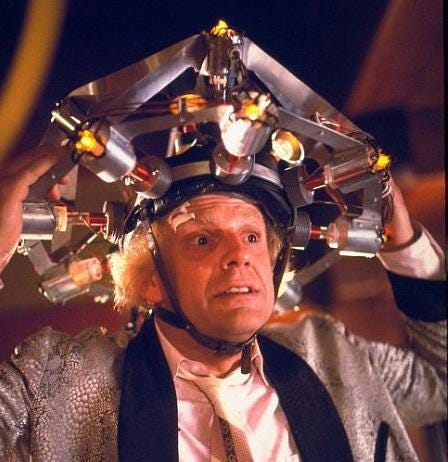-=+=- -=+=- -=+=- -=+=- -=+=- -=+=- -=+=- -=+=- -=+=- -=+=- -=+=- -=+=- -=+=- -=+=- -=+=- -=+=- -=+=- -=+=- -=+=- -=+=- -=+=- -=+=- -=+=- -=+=- -=+=- -=+=- -=+=- -=+=- -=+=- -=+=- (c) WidthPadding Industries 1987 0|356|0 -=+=- -=+=- -=+=- -=+=- -=+=- -=+=- -=+=- -=+=- -=+=- -=+=- -=+=- -=+=- -=+=- -=+=- -=+=- -=+=- -=+=- -=+=- -=+=- -=+=- -=+=- -=+=- -=+=- -=+=- -=+=- -=+=- -=+=- -=+=- -=+=- -=+=- Socoder -> Off Topic -> The Robots Are Not Attacking (Yet) Posted : Tuesday, 26 June 2018, 13:03 DabzNo escape: Linkage-=-=- Intel Core i5 6400 2.7GHz, NVIDIA GeForce GTX 1070 (8GB), 8Gig DDR4 RAM, 256GB SSD, 1TB HDD, Windows 10 64bitPosted : Tuesday, 26 June 2018, 13:03 DanMaybe, if you wear this:Posted : Tuesday, 26 June 2018, 21:40 PakzNot so many years from now(I hope) machines will be able to solve complex problems much like a calculator can solve a complex calculation. Those neural networks systems do have taken off but the power of computers still is not really good yet. I watched a video where they said that for a computer to be at the same level of the human brain would require the energy of a nuclear power plant. There was news yesterday that they had a computer player for Dota? I believe that could beat human players with more simplified game rules. They needed to train it on more than a hundred thousand cpu's iirc. I have been letting a couple of my laptops work for stanfords folding at home project for a couple of days every month for 8 months now. Still going at the same project all that time. This stanford network is one of the most powerfull in the world. Progress is still slow I think. edit: Myself I tried to do a brute force pathfinding algorithm and with more then 6 steps deep it struggles on my 7th gen i5.